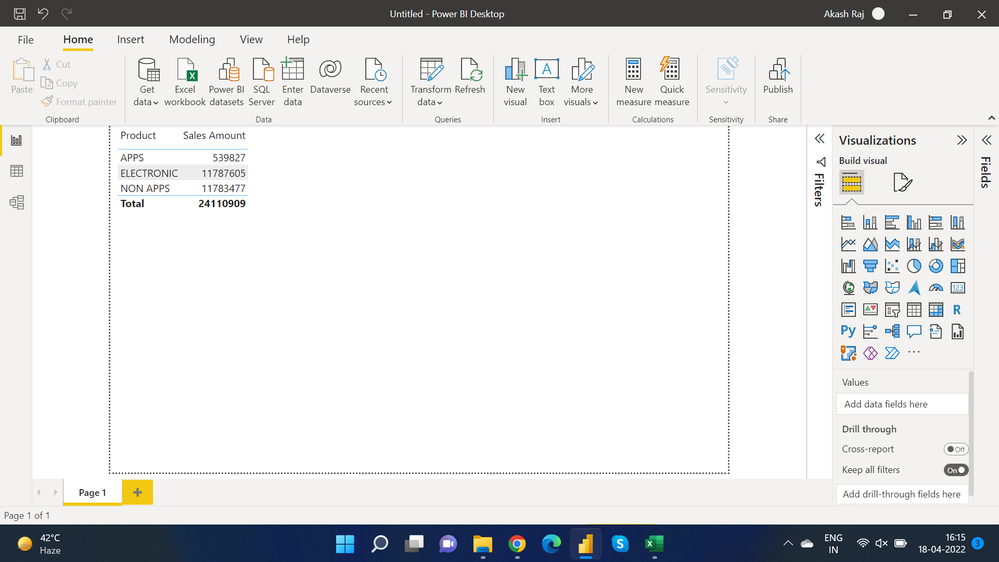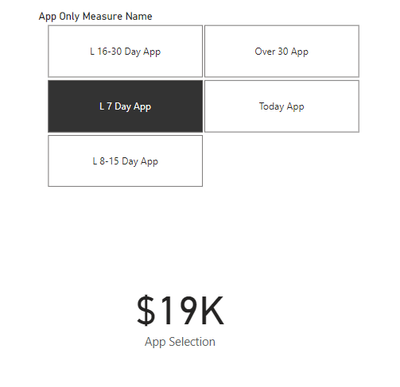cancel
Showing results for
Did you mean:Frequent Visitor

## Sales Between Two Days, Not Date.

Please find screenshot for better understanding,Issue Is:

I have to calculate the sum of Sales Amount column only for two product i.e "APPS" And "NONAPPS", For Today, For Last 7 Days, For Last 8 To 15 Days, For Last 15 To 30 Days, More Than 30 Days.

Today Means - Sum Of Today i.e 18-04-2022 Sales For APPS And NONAPPS Product category.

Last 7 Days Means - Sum Of Sales Between Yesterday i.e. 17-04-2022 To 7 Days Back i.e. 11-04-2022, For APPS And NONAPPS Product category.

Last 8 To 15 Days Means - Sum Of Sales Between 10-04-2022 To 27-03-2022, For APPS And NONAPPS Product category.

Last 16 To 30 Days Means - Sum Of Sales Between 26-03-2022 To 12-03-2022, For APPS And NONAPPS Product category.

More Than 30 Days Means - Sum Of Sales Happened Before 12-03-2022.

Dataset Is Attached.

Measures Has To Be Individual So Total 5 Measures I Have To Create, Please Help Me Community And Experts.

1 ACCEPTED SOLUTIONCommunity Support

Hi, @it_akasjraj

You can try the following methods. Because I replied to your post on April 21, I subtracted three days from each today date to get the date of your original post on April 18.

Measure:

``````Today =
CALCULATE(SUM('Table'[Sales Amount]),FILTER(ALL('Table'),[Product]="APPS"&&[Date]=TODAY()-3))
+CALCULATE(SUM('Table'[Sales Amount]),FILTER(ALL('Table'),[Product]="NON APPS"&&[Date]=TODAY()-3))``````
``````Last 7 Days =
CALCULATE(SUM('Table'[Sales Amount]),FILTER(ALL('Table'),[Product]="APPS"&&[Date]<=TODAY()-3-1&&[Date]>=TODAY()-3-7))
+CALCULATE(SUM('Table'[Sales Amount]),FILTER(ALL('Table'),[Product]="NON APPS"&&[Date]<=TODAY()-3-1&&[Date]>=TODAY()-3-7))``````
``````Last 8 To 15 Days =
CALCULATE(SUM('Table'[Sales Amount]),FILTER('Table',[Product]="APPS"&&[Date]<=TODAY()-3-8&&[Date]>=TODAY()-3-8-14))
+CALCULATE(SUM('Table'[Sales Amount]),FILTER('Table',[Product]="NON APPS"&&[Date]<=TODAY()-3-8&&[Date]>=TODAY()-3-8-14))``````
``````Last 16 To 30 Days =
CALCULATE(SUM('Table'[Sales Amount]),FILTER(ALL('Table'),[Product]="APPS"&&[Date]<=TODAY()-3-23&&[Date]>=TODAY()-3-37))
+CALCULATE(SUM('Table'[Sales Amount]),FILTER(ALL('Table'),[Product]="NON APPS"&&[Date]<=TODAY()-3-23&&[Date]>=TODAY()-3-37))``````
``````More Than 30 Days =
CALCULATE(SUM('Table'[Sales Amount]),FILTER(ALL('Table'),[Product]="APPS"&&[Date]<TODAY()-3-37))
+CALCULATE(SUM('Table'[Sales Amount]),FILTER(ALL('Table'),[Product]="NON APPS"&&[Date]<TODAY()-3-37))``````Is this what you expect? It can be compared with the data you expect.

Best Regards,

Community Support Team _Charlotte

If this post helps, then please consider Accept it as the solution to help the other members find it more quickly.

4 REPLIES 4Super User

Hi:

When you upload the file you will see all three broken out by period.

App & Non-App

App

Non-App

For instance if you choose Last 7 Days:Super User

Hi:

I added a few days to your dataset to bring to today. There is a Date Table with a Day Index on the attached file. This was used along with a measure selector table to obtain results. Should make it easy for user to select:-)

I hope this helps.Community Support

Hi, @it_akasjraj

You can try the following methods. Because I replied to your post on April 21, I subtracted three days from each today date to get the date of your original post on April 18.

Measure:

``````Today =
CALCULATE(SUM('Table'[Sales Amount]),FILTER(ALL('Table'),[Product]="APPS"&&[Date]=TODAY()-3))
+CALCULATE(SUM('Table'[Sales Amount]),FILTER(ALL('Table'),[Product]="NON APPS"&&[Date]=TODAY()-3))``````
``````Last 7 Days =
CALCULATE(SUM('Table'[Sales Amount]),FILTER(ALL('Table'),[Product]="APPS"&&[Date]<=TODAY()-3-1&&[Date]>=TODAY()-3-7))
+CALCULATE(SUM('Table'[Sales Amount]),FILTER(ALL('Table'),[Product]="NON APPS"&&[Date]<=TODAY()-3-1&&[Date]>=TODAY()-3-7))``````
``````Last 8 To 15 Days =
CALCULATE(SUM('Table'[Sales Amount]),FILTER('Table',[Product]="APPS"&&[Date]<=TODAY()-3-8&&[Date]>=TODAY()-3-8-14))
+CALCULATE(SUM('Table'[Sales Amount]),FILTER('Table',[Product]="NON APPS"&&[Date]<=TODAY()-3-8&&[Date]>=TODAY()-3-8-14))``````
``````Last 16 To 30 Days =
CALCULATE(SUM('Table'[Sales Amount]),FILTER(ALL('Table'),[Product]="APPS"&&[Date]<=TODAY()-3-23&&[Date]>=TODAY()-3-37))
+CALCULATE(SUM('Table'[Sales Amount]),FILTER(ALL('Table'),[Product]="NON APPS"&&[Date]<=TODAY()-3-23&&[Date]>=TODAY()-3-37))``````
``````More Than 30 Days =
CALCULATE(SUM('Table'[Sales Amount]),FILTER(ALL('Table'),[Product]="APPS"&&[Date]<TODAY()-3-37))
+CALCULATE(SUM('Table'[Sales Amount]),FILTER(ALL('Table'),[Product]="NON APPS"&&[Date]<TODAY()-3-37))``````Is this what you expect? It can be compared with the data you expect.

Best Regards,

Community Support Team _Charlotte

If this post helps, then please consider Accept it as the solution to help the other members find it more quickly.Super User

no need to create 5 measures.
You can create a disconnected table that contains the Day values:

Selection                  Value

Today                    0

Last 7 Days            7

etc.

you can do it in excel or any way makes comfortable.
Use this table to create a slicer or drag in to your matix columns then modify your measure to adapt for SELECTEDVALUE instead of hard coding a fixed number for each measure